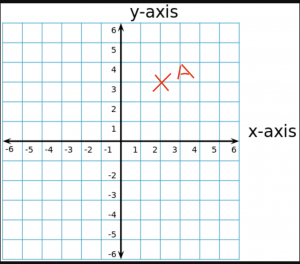Online Math Center

# All You Need To Know About Coordinate Planes

In 6th grade, students start to learn geometrical planes and how to envision different shapes and constructions in 2D. Developing a 2D vision enables students to understand architecture and translate it, later on, into 3D representations. The coordinate plane system is hundred years old and was invented by Rene Descartes, and it was primarily called The Cartesian Plan. The fundamental 2D representation of shapes is constructed on coordinate planes, so let’s see what this means.

## Definition and Representation of Coordinate Planes

A coordinate plane is a two-dimensional representation formed by two intersecting, perpendicular lines. The two lines are named: X-axis being the horizontal line, Y-axis being the vertical line.

Anywhere we choose a point on the coordinate plane, that point will have two indicators (x-axis, y-axis). As we can see from the image above, the left side of the x or y-axis has negative values, therefore any number on the bottom, left-part will be a negative integer. The middle point, the intersection of the two axes is called the origin or 0.

The purpose of a coordinate plane is to allow the sketching or planning of different objects, or shapes within certain measurements. It is like a template or a canvas, a map of shapes.

## Elements Of The Coordinate Plane

A coordinate plane is built by 4 quadrants, indicated by the intersection of the two axes. As seen in the image above when X-axis intersects the Y-axis, the result is four different areas called quadrants, indicated by roman numerals:Quadrant I: Both axes, the X-axis and Y-axis have positive numbers, in the upper right area.

Quadrant II: X-axis has negative numbers, and Y-axis has positive numbers, in the upper left area.

Quadrant III: Both axes, the X-axis and Y-axis have negative numbers, in the left bottom area;

Quadrant IV: Y-axis has negative numbers, and X-axis has positive numbers, in the right bottom area;

Coordinate: points in the coordinate plane are indicated by pair of X and Y indicators, always mapped by the  (X, Y) pair.

For example, let’s take point A:On the coordinate plane, point A is given by the pair (2, 3).

Try to indicate the following points on the coordinate plane. Remember that the point is given in the order X and Y (X, Y).

A1 (3, 4) D (5, 6)

B ( -1, 2) E ( -4, 1)

C ( – 5, – 2) F ( 0, 0)

We can also represent all the points on the X-axis with (X, 0), while all the points on the Y-axis are represented by (0, Y).

Do you understand the geometry of the coordinate plane system? You can test the infinite aspect of the coordinate plan system online, here

1. In which quadrants of the coordinate plane are the following points?
• A (2, – 2 )
• B (4, – 5)
• C (- 2,-3)
• D (- 1, 1)

1.  Which of the following points lie on the x-axis? Indicate your answers by drawing the coordinate plane and the given coordinates:

• A ( – 6, 0)
• B (0, 2)
• C ( -3, 0)
• D ( 5, 0)

Are you ready to learn more about geometrical planes? The OMC math tutors offer a wide range of math lessons for middle school students. Our programs are also designed to prepare students for math competitions. Reach out to us and discover more fun facts about maths!

## Step One

It only takes two steps to schedule a free lesson with an OMC representative.

To get started, please tell us who you are:

## Dear Parent!

Thank you for placing your trust in us to educate your child!

Congratulations on joining the Online Math Center!
See you in class.

## Thank you!

Our manager will be in contact with you shortly.

## Step Two

Let us know how to contact you. One of our representatives will get back to you shortly.

## Step Two

Awesome! We need to get in touch with your parent or guardian for further discussion. Please check in with them before filling out the form below with their information.

## Step One

It only takes two steps to schedule an appointment with an OMC representative.

To get started, please tell us who you are: